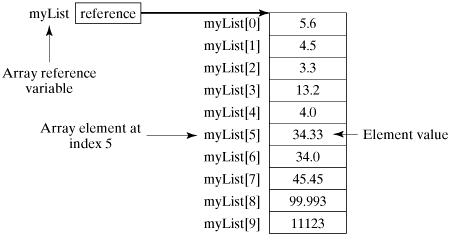# java怎么定义数组？Java中定义数组的方法

TheDisguiser 2020-03-29 17:07:32 java常见问答 5240

Java是一门博大精深的语言，用Java做软件开发一定离不开数组，那么大家知道数组吗?在Java中又要怎么定义数组呢?今天小编就来教大家如何在Java中定义及使用数组。

1.声明数组变量

```dataType[] arrayRefVar; // 首选的方法 或 dataType arrayRefVar[]; //

`double[] myList; // 首选的方法 或 double myList[]; // 效果相同，但不是首选方法`

Java语言使用new操作符来创建数组，语法如下：

`arrayRefVar = new dataType[arraySize];`

`dataType[] arrayRefVar = new dataType[arraySize];`

`dataType[] arrayRefVar = {value0, value1, ..., valuek};`

TestArray.java 文件代码：

```public class TestArray {
public static void main(String[] args) {
// 数组大小
int size = 10;
// 定义数组
double[] myList = new double[size];
myList = 5.6;
myList = 4.5;
myList = 3.3;
myList = 13.2;
myList = 4.0;
myList = 34.33;
myList = 34.0;
myList = 45.45;
myList = 99.993;
myList = 11123;
// 计算所有元素的总和
double total = 0;
for (int i = 0; i < size; i++) {
total += myList[i];
}
System.out.println("总和为： " + total);
}
}```

`总和为： 11367.373`TestArray.java 文件代码：

```public class TestArray {
public static void main(String[] args) {
double[] myList = {1.9, 2.9, 3.4, 3.5};
// 打印所有数组元素
for (int i = 0; i < myList.length; i++) {
System.out.println(myList[i] + " ");
}

// 计算所有元素的总和 double total = 0;
for (int i = 0; i < myList.length; i++) {
total += myList[i];
}
System.out.println("Total is " + total);

// 查找最大元素 double max = myList;
for (int i = 1; i < myList.length; i++) {
if (myList[i] > max) max = myList[i];
}
System.out.println("Max is " + max);
}
}```

`1.92.93.43.5Total is 11.7Max is 3.5`

For-Each 循环

JDK 1.5 引进了一种新的循环类型，被称为 For-Each 循环或者加强型循环，它能在不使用下标的情况下遍历数组。

```for(type element: array){
System.out.println(element);
}```

```public class TestArray {
public static void main(String[] args) {
double[] myList = {1.9, 2.9, 3.4, 3.5};
// 打印所有数组元素
for (double element: myList) {
System.out.println(element);
}
}
}```

`1.92.93.43.5`

```public static void printArray(int[] array) {
for (int i = 0; i < array.length; i++) {
System.out.print(array[i] + " ");
}
}```

`printArray(new int[]{3, 1, 2, 6, 4, 2});`

```public static int[] reverse(int[] list) {
int[] result = new int[list.length];
for (int i = 0, j = result.length - 1; i < list.length; i++, j--) {
result[j] = list[i];
}
return result;
}```

`String str[][] = new String;`

1. 直接为每一维分配空间，格式如下：

`type[][] typeName = new type[typeLength1][typeLength2];`

type 可以为基本数据类型和复合数据类型，arraylength1 和 arraylength2 必须为正整数，arraylength1 为行数，arraylength2 为列数。

`int a[][] = new int;`

2. 从最高维开始，分别为每一维分配空间，例如：

```String s[][] = new String[];
s = new String;
s = new String;
s = new String("Good");
s = new String("Luck");
s = new String("to");
s = new String("you");
s = new String("!");```

s=new String 和 s=new String 是为最高维分配引用空间，也就是为最高维限制其能保存数据的最长的长度，然后再为其每个数组元素单独分配空间 s0=new String("Good") 等操作。

`num;`

Arrays 类

java.util.Arrays 类能方便地操作数组，它提供的所有方法都是静态的。